# Texas Go Math Grade 3 Lesson 10.3 Answer Key Division Models

Refer to our Texas Go Math Grade 3 Answer Key Pdf to score good marks in the exams. Test yourself by practicing the problems from Texas Go Math Grade 3 Lesson 10.3 Answer Key Division Models.

## Texas Go Math Grade 3 Lesson 10.3 Answer Key Division Models

Essential Question
How can you use strip diagrams to solve division problems?
Strip diagram is used like counters and circle type of diagram.
Explanation:
For example;
24 books equally divided into 6 in 4 groups.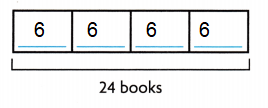Unlock the problem
A dog trainer has 20 dog treats for 5 dogs in his class. If each dog gets the same number of treats, how many treats will each dog get?
4 treats
Explanation:
A dog trainer has 20 dog treats for 5 dogs in his class.
If each dog gets the same number of treats,
Number of treats each dog get
20 ÷ 5 = 4

• What do you need to find?
Number of treats will each dog get.

Activity 1
Use counters to find how many in each group.
Materials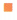countersMath Board

• Use 20 counters.
• Draw 5 circles on your Math Board.
• Place 1 counter at a time in each circle until all 20 counters are used.
• Draw the rest of the counters to show your work.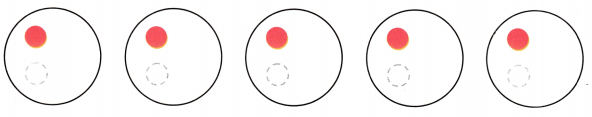There are ___ counters in each of the 5 groups.
There are 5 counters in each of the group.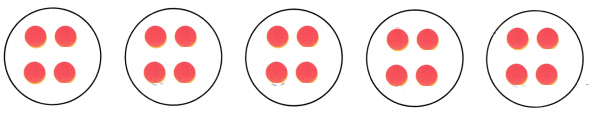A strip diagram can show how the parts of a problem are related.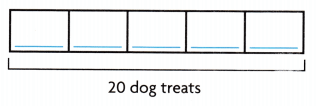• Complete the strip diagram to show 20 dog treats divided into 5 equal groups.
So, each dog will get ___ treats.
Each dog will get 5 treats.Explanation:
A dog trainer has 20 dog treats for 5 dogs in his class.
If each dog gets the same number of treats,
Number of treats each dog get
20 ÷ 5 = 4

Activity 2
Draw to find how many equal groups.

A dog trainer has 20 dog treats. If he gives 5 treats to each dog in his class, how many dogs are in the class?• Look at the 20 counters.
• Circle a group of 5 counters.
• Continue circling groups of 5 until all 20 counters are in groups.
There are ___ groups of 5 counters.
• Complete the strip diagram to show 20 treats divided into groups of 5 treats.So, there are __ dogs in the class.
So, there are 4 dogs in the class.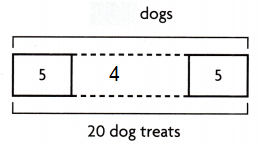An equation is a number sentence that uses the equal sign to show that two amounts are equal.
Here are two ways to record division.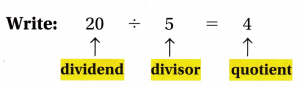Read: Twenty divided by five equals four.Share and Show

Question 1.
Complete the picture to find 12 ÷ 4.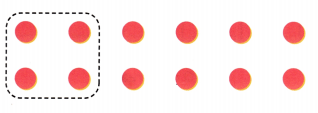Math Talk
Mathematical Processes
Explain how you know to make.
By using counters, and putting the same amount into each group then we count each group. The equal groups model used in multiplication can be used to solve division problems as well.
Understand division as an unknown-factor problem.

Complete the strip diagram to solve. Then write a division equation for the strip diagram.

Question 2.
There are 24 books in 4 equal stacks. ‘ How many books are in each stack?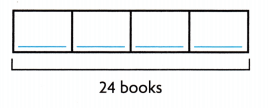6 booksExplanation:
There are 24 books in 4 equal stacks.
Number of books in each stack
24 ÷ 4 = 6

There are 8 matching socks. How many pairs of socks can you make?4 pairs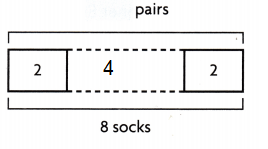Explanation:
There are 8 matching socks.
Total pairs of socks can make
8 ÷ 2 = 4

Problem Solving

Use the table for 4-5.

Question 4.
H.O.T. Multi-Step Kevin bought a box of Puppy Chips for his dogs. If he gives his small dog 2 treats each day and his big dog 4 treats each day, for how many days will one box of treats last?7 days.
Explanation:
Kevin bought a box of Puppy Chips for his dogs.
Number of puppy chips in each box = 42
If he gives his small dog 2 treats each day and his big dog 4 treats each day,
Total puppy chips each day = 2 + 4 = 6
Number of days one box of treats last
42 ÷ 6 = 7

Question 5.
H.O.T. Multi-Step Pat bought one box of Chew Sticks to share equally between his 2 dogs. Mia bought one box of Chewies to share equally among her 5 dogs. How many more treats will each of Pat’s dogs get than each of Mia’s dogs? Explain.
Each dog gets 2 more treats
Explanation:
1 box of chew sticks contain 14
Pat bought one box of Chew Sticks to share equally between his 2 dogs.
14 ÷  2 = 7
1 box of chewies contain 25
Mia bought one box of Chewies to share equally among her 5 dogs.
25 ÷ 5 = 5
Number of more treats each of Pat’s dogs get than each of Mia’s dogs
7 – 5 = 2

Fill in the bubble for the correct answer choice.
You may use models or strategies to solve.

Question 6.
Multi-Step A day on Earth is 24 hours long. A day on the dwarf planet Eris is 8 hours long. How many Eris days are equal to 2 Earth days?
(A) 16
(B) 6
(C) 4
(D) 40
Option (B)
Explanation:
A day on Earth is 24 hours long.
2 Earth days is 24 x 2 = 48
A day on the dwarf planet Eris is 8 hours long.
Number of Eris days equal to 2 Earth days
48 ÷ 8 = 6

Question 7.
Jaclyn uses 21 cups of flour to make bread. Each loaf of bread takes 3 cups of flour. How many loaves of bread is Jaclyn making?
(A) 8
(B) 18
(C) 24
(D) 7
Option (D)
Explanation:
Jaclyn uses 21 cups of flour to make bread.
Each loaf of bread takes 3 cups of flour.
Total loaves of bread Jaclyn making
21 ÷ 3 = 7

Multi-Step Ryan has a package of striped mini-kites that he divides equally between 2 friends. Kara has a box of rainbow mini-kites that she divides equally among 4 friends. How many more mini-kites does each Striped
Ryan’s friends get more than each of Kara’s friends.(A) 1
(B) 9
(C) 5
(D) 4
Option (A)
Explanation:
Ryan has a package of striped mini-kites that he divides equally between 2 friends.
1 package of striped mini kites is 10
So, 10 ÷ 2 = 5
Kara has a box of rainbow mini-kites that she divides equally among 4 friends.
1 package of rainbow kites is 16
So, 16 ÷ 4 = 4
1 more mini-kites than each of Striped kites.

Texas Test Prep

Question 9.
Use a Diagram Ed buys 5 bags of treats. He buys 15 treats in all. How many treats are in each bag?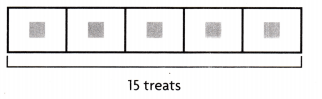(A) 5
(B) 3
(C) 4
(D) 2
Option (B)
Explanation:
Ed buys 5 bags of treats.
He buys 15 treats in all.
Total treats in each bag
15 ÷ 5 = 3

### Texas Go Math Grade 3 Lesson 10.3 Homework and Practice Answer Key

Complete the strip diagram to solve. Then write a division equation for the strip diagram.

Question 1.
Alice has 25 dimes in 5 equal stacks. How many dimes are in each stack?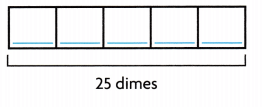5 dimes in each stack
Explanation:Alice has 25 dimes in 5 equal stacks.
Total dimes in each stack
25 ÷ 5 = 5

Question 2.
Toby has 12 action figures in sets of 3. How many sets does Toby have?Toby has 4 sets
Explanation:Toby has 12 action figures in sets of 3.
Number of sets Toby have
12 ÷ 3 = 4

Problem Solving

Use the table for 3-6.

Question 3.
If a gardener plants the rose bushes into 7 equal rows, how many bushes are in each row?5 rose bushes in each row.
Explanation:
If a gardener plants the rose bushes into 7 equal rows,
Number of rose bushes 35
Number of bushes in each row
35 ÷ 7 = 5

Question 4.
If Mia puts the box plants into 3 equal rows, how many plants will be in each row?
7 plants in each row.
Explanation:
If Mia puts the box plants into 3 equal rows,
Number of box plants 21
Total plants in each row
21 ÷ 3 = 7

Question 5.
Lawrence arranges the azalea and box plants into 6 equal rows. How many plants are in each row?
6 plants in each row.
Explanation:
Lawrence arranges the azalea and box plants into 6 equal rows.
Number of Azalea 15
Number of box plants 21
Total number of Azalea and box plants 15 + 21 = 36
Total plants in each row
36 ÷ 6 = 6

Question 6.
Mrs. Kimura wants to put an equal number of azalea plants and lilac bushes into 5 equal rows. How many plants will be in each row?
8 plants in each row.
Explanation:
Mrs. Kimura wants to put an equal number of azalea plants and lilac bushes into 5 equal rows.
Number of Azalea plants 15
Number of Lilac bushes 25
Total number of Azalea and Lilac bushes 15 + 25 = 40
Number of plants in each row
40 ÷ 5 = 8

Texas Test Prep

Question 7.
Ethan has 24 baseball cards that he divides among himself and 3 friends. How many cards does each person get?
(A) 6
(B) 8
(C) 21
(D) 27
Option(B)
Explanation:
Ethan has 24 baseball cards that he divides among himself and 3 friend
The total cards each person gets
24 ÷ 3 = 8

Beth sews an equal number of buttons onto 6 puppets. If she has 42 buttons, how many buttons does each puppet get?
(A) 9
(B) 6
(C) 7
(D) 8
Option(C)
Explanation:
Beth sews an equal number of buttons onto 6 puppets.
If she has 42 buttons,
Number of buttons each puppet get
42 ÷ 6 = 7

Question 9.
Susi buys 4 boxes of granola bars. She buys 20 bars in all. How many granola bars are in each box?
(A) 16
(B) 5
(C) 4
(D) 24
Option(B)
Explanation:
Susi buys 4 boxes of granola bars.
She buys 20 bars in all.
Number of granola bars in each box
20 ÷ 4 = 5

Question 10.
A group of 35 scouts go on a camping trip. If the scouts sleep in tents in groups of 5, how many tents will they need?
(A) 8
(B) 7
(C) 30
(D) 9
Option(B)
Explanation:
A group of 35 scouts go on a camping trip.
If the scouts sleep in tents in groups of 5,
Number of many tents they need
35 ÷ 5 = 7

Question 11.
Multi-Step Ms. McCall divides 24 crayons among her 4 students. Then, she divides 28 markers among her 4 students. How many more markers will each of the students get than crayons?
(A) 4
(B) 2
(C) 1
(D) 10
Option(C)
Explanation:
Ms. McCall divides 24 crayons among her 4 students.
24 ÷ 4 = 6
Then, she divides 28 markers among her 4 students.
28 ÷ 4 = 7
Number of more markers each of the students get than crayons
7 – 6 = 1

Question 12.
Multi-Step There are 27 plums divided equally in 3 bowls and 35 apricots divided equally into 7 bowls. How many more plums than apricots are there in one bowl of each?
(A) 12
(B) 3
(C) 5
(D) 4